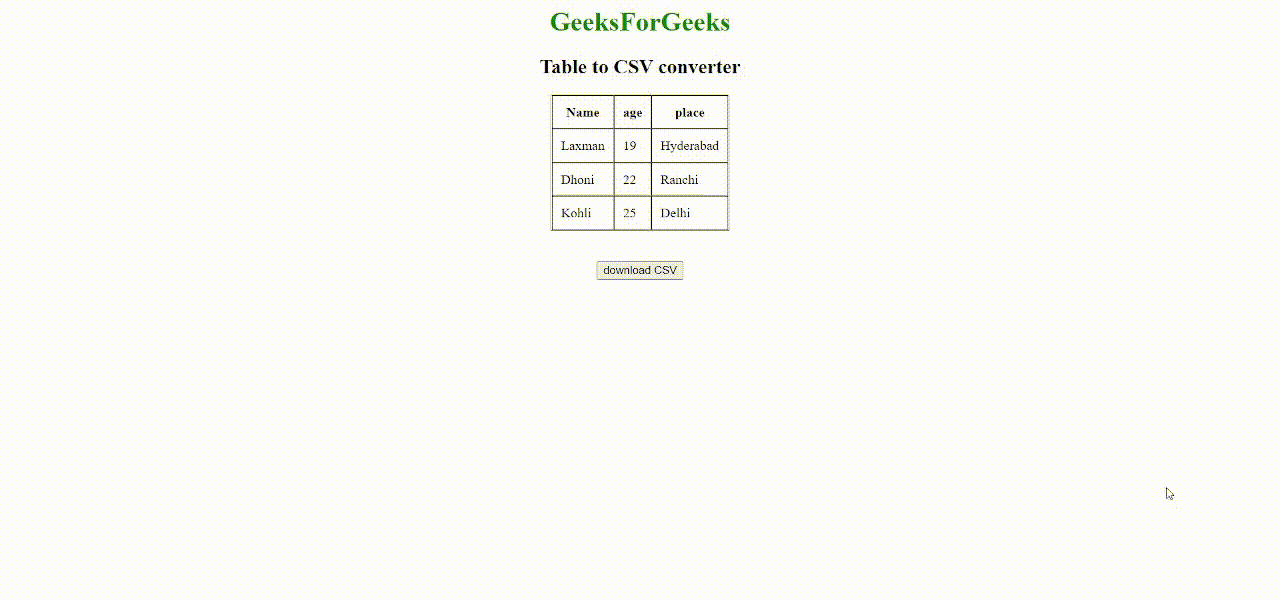GFG App
Open AppBrowser
Continue

# How to export HTML table to CSV using JavaScript ?

Comma Separated Values or CSV is a type of text file where each value is delimited by a comma. CSV files are very useful for the import and export of data to other software applications.

Sometimes while developing web applications, you may come into a scenario where you need to download a CSV file converted from an HTML table. In this post, let us discuss how to create this feature using pure JavaScript without using any fancy plugins, modules, or frameworks.

Approach: Create an HTML table. By using JavaScript and Document object module (DOM), we are going to extract each column data in a row and combine the data using commas. After doing this to each row, again using DOM, we are going to create a new download link and trigger the link using JavaScript event listeners to download the data to form a CSV file.

Step 1: Create an HTML table: Create a simple HTML page with a table and a button. This button will be used as a trigger to convert the table into comma-separated values and download it in the form of a CSV file. Apply your own needed CSS stylings.

## HTML

 `` `<``html``>`   `<``body``>` `    ``<``center``>` `        ``<``h1` `style``=``"color:green"``>GeeksForGeeks` `        ``<``h2``>Table to CSV converter` `        ``<``table` `border``=``"1"` `cellspacing``=``"0"` `cellpadding``=``"10"``>` `            ``<``tr``>` `                ``<``th``>Name` `                ``<``th``>age` `                ``<``th``>place` `            ``` `            ``<``tr``>` `                ``<``td``>Laxman` `                ``<``td``>19` `                ``<``td``>Hyderabad` `            ``` `            ``<``tr``>` `                ``<``td``>Dhoni` `                ``<``td``>22` `                ``<``td``>Ranchi` `            ``` `            ``<``tr``>` `                ``<``td``>Kohli` `                ``<``td``>25` `                ``<``td``>Delhi` `            ``` `        ``` `        ``<``br``><``br``>` `        ``<``button` `type``=``"button"``>download CSV` `    ``` ``   ``

Step 2: Convert table data into comma-separated values: Write a JavaScript function to retrieve the table data and convert it to comma-separated values. Make use of the document object model to access table data in each column of the rows. This function should be triggered when the user clicks the download button.

## Javascript

 `function` `tableToCSV() {`   `    ``// Variable to store the final csv data` `    ``var` `csv_data = [];`   `    ``// Get each row data` `    ``var` `rows = document.getElementsByTagName(``'tr'``);` `    ``for` `(``var` `i = 0; i < rows.length; i++) {`   `        ``// Get each column data` `        ``var` `cols = rows[i].querySelectorAll(``'td,th'``);`   `        ``// Stores each csv row data` `        ``var` `csvrow = [];` `        ``for` `(``var` `j = 0; j < cols.length; j++) {`   `            ``// Get the text data of each cell of` `            ``// a row and push it to csvrow` `            ``csvrow.push(cols[j].innerHTML);` `        ``}`   `        ``// Combine each column value with comma` `        ``csv_data.push(csvrow.join(``","``));` `    ``}` `    ``// combine each row data with new line character` `    ``csv_data = csv_data.join(``'\n'``);`   `    ``/* We will use this function later to download` `    ``the data in a csv file downloadCSVFile(csv_data);` `    ``*/` `}`

When the tableToCSV() function is triggered, it accesses each table row data using the document object model. The getElementByTagName(‘tr’) retrieves all table row data and stores it in rows variable. The rows[i].querySelectorAll(‘td,th’) will get each column data of that table row. It is then stored in csvrow variable. The csvrow variable data are combined using commas to represent a row of a CSV file and then it is stored in a csv_data variable which represents the data of our CSV file in combination. We then join csv_data values using the newline character as each row in a CSV file is represented in a new line. Now our data is ready to be exported into a CSV file.

Step 3: Write a script to download the CSV file: Now that we have our converted data ready, we need to write a script to create a CSV file, feed our data into it, and trigger the browser to download it automatically after the user has clicked the download button. Since this function will be triggered after the table data is converted, we will call this function inside tableToCSV() function.

## Javascript

 `function` `downloadCSVFile(csv_data) {`   `    ``// Create CSV file object and feed our` `    ``// csv_data into it` `    ``CSVFile = ``new` `Blob([csv_data], { type: ``"text/csv"` `});`   `    ``// Create to temporary link to initiate` `    ``// download process` `    ``var` `temp_link = document.createElement(``'a'``);`   `    ``// Download csv file` `    ``temp_link.download = ``"GfG.csv"``;` `    ``var` `url = window.URL.createObjectURL(CSVFile);` `    ``temp_link.href = url;`   `    ``// This link should not be displayed` `    ``temp_link.style.display = ``"none"``;` `    ``document.body.appendChild(temp_link);`   `    ``// Automatically click the link to trigger download ` `    ``temp_link.click();` `    ``document.body.removeChild(temp_link);` `}`

Final Code:

## HTML

 `` `<``html``>`   `<``body``>` `    ``<``center``>` `        ``<``h1` `style``=``"color:green"``>GeeksForGeeks` `        ``<``h2``>Table to CSV converter` `        ``<``table` `border``=``"1"` `cellspacing``=``"0"` `cellpadding``=``"10"``>` `            ``<``tr``>` `                ``<``th``>Name` `                ``<``th``>age` `                ``<``th``>place` `            ``` `            ``<``tr``>` `                ``<``td``>Laxman` `                ``<``td``>19` `                ``<``td``>Hyderabad` `            ``` `            ``<``tr``>` `                ``<``td``>Dhoni` `                ``<``td``>22` `                ``<``td``>Ranchi` `            ``` `            ``<``tr``>` `                ``<``td``>Kohli` `                ``<``td``>25` `                ``<``td``>Delhi` `            ``` `        ``` `        ``<``br``><``br``>` `        ``<``button` `type``=``"button"` `onclick``=``"tableToCSV()"``>` `            ``download CSV` `        ``` `    ```   `    ``<``script` `type``=``"text/javascript"``>` `        ``function tableToCSV() {`   `            ``// Variable to store the final csv data` `            ``var csv_data = [];`   `            ``// Get each row data` `            ``var rows = document.getElementsByTagName('tr');` `            ``for (var i = 0; i < ``rows.length``; i++) {`   `                ``// Get each column data` `                ``var ``cols` `= ``rows``[i].querySelectorAll('td,th');`   `                ``// Stores each csv row data` `                ``var csvrow = [];` `                ``for (var ``j` `= ``0``; j < cols.length; j++) {`   `                    ``// Get the text data of each cell` `                    ``// of a row and push it to csvrow` `                    ``csvrow.push(cols[j].innerHTML);` `                ``}`   `                ``// Combine each column value with comma` `                ``csv_data.push(csvrow.join(","));` `            ``}`   `            ``// Combine each row data with new line character` `            ``csv_data` `= csv_data.join('\n');`   `            ``// Call this function to download csv file  ` `            ``downloadCSVFile(csv_data);`   `        ``}`   `        ``function downloadCSVFile(csv_data) {`   `            ``// Create CSV file object and feed` `            ``// our csv_data into it` `            ``CSVFile` `= ``new` `Blob([csv_data], {` `                ``type: "text/csv"` `            ``});`   `            ``// Create to temporary link to initiate` `            ``// download process` `            ``var ``temp_link` `= ``document``.createElement('a');`   `            ``// Download csv file` `            ``temp_link.download` `= ``"GfG.csv"``;` `            ``var ``url` `= ``window``.URL.createObjectURL(CSVFile);` `            ``temp_link.href` `= ``url``;`   `            ``// This link should not be displayed` `            ``temp_link.style.display` `= ``"none"``;` `            ``document.body.appendChild(temp_link);`   `            ``// Automatically click the link to` `            ``// trigger download` `            ``temp_link.click();` `            ``document.body.removeChild(temp_link);` `        ``}` `    ``` ``   ``

Output:Html table to CSV file

My Personal Notes arrow_drop_up